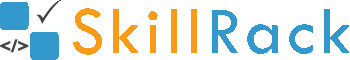Various ways to swap two numbers (integers) 1 year ago

The following are the commonly used logic to swap two values.

Approach 1: Swap using third (temporary) variable

#include <stdio.h>

int main(){
int x,y;
scanf("%d%d",&x,&y);
int temp = x;
x = y;
y = temp;
printf("%d %d",x,y);
}

Approach 2: Swap using XOR operator

#include <stdio.h>

int main(){
int x,y;
scanf("%d%d",&x,&y);
x = x^y;
y = x^y; //Now y = (x^y)^y = x. So y has value of x now
x = x^y; //Now x = (x^y)^x = x
printf("%d %d",x,y);
}

Approach 3: Swap using Addition and Subtraction

#include <stdio.h>

int main(){
int x,y;
scanf("%d%d",&x,&y);
x = x+y; //Assume x=5 and y=3. Now x=8, y=3
y = x-y; //Now x=8 and y=5
x = x-y; //Now x=3 and y=5 and hence swapped.
printf("%d %d",x,y);
}

Approach 4: Swap using Multiplication and Division

#include <stdio.h>

int main(){
int x,y;
scanf("%d%d",&x,&y);
x = (x*y)/(y=x);
printf("%d %d",x,y);
}